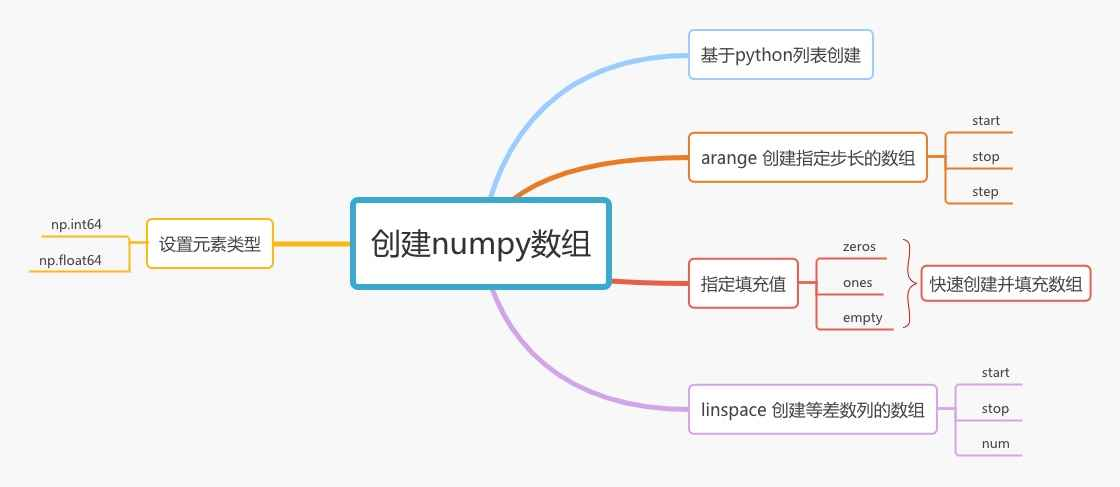# 第3节，如何创建numpy数组1. 基于列表
2. zeros, ones, empty 指定填充值
3. arange 创建指定步长的数组
4. linspace 创建等差数列的数组

### 1. 基于python列表创建

``````import numpy as np

a = np.array([1, 2, 3])
print(a)        # [1 2 3]
``````

``````import numpy as np

a = np.array([[1, 2, 3], [4, 5, 6], [7, 8, 9]])
print(a)
print(a.ndim)       # 2  2维数组
print(a.shape)      # (3, 3)
``````

### 2. zeros, ones, empty 指定填充值

zeros方法创建一个ndarray，数组中的元素值都是0，ones方法创建一个ndarray，数组中的元素值都是1

``````import numpy as np

# 创建一维数组
zero_array = np.zeros(3)
print(zero_array)

one_array = np.ones(5)
print(one_array)

# 创建二维数组
zero_array_2d = np.zeros((3, 4))   # 3行4列
print(zero_array_2d)

one_array_2d = np.ones((5, 2))   # 5行2列
print(one_array_2d)
``````

``````[0. 0. 0.]

[1. 1. 1. 1. 1.]

[[0. 0. 0. 0.]
[0. 0. 0. 0.]
[0. 0. 0. 0.]]

[[1. 1.]
[1. 1.]
[1. 1.]
[1. 1.]
[1. 1.]]
``````

zeros方法的第一个参数shape可以是一个int， 也可以是一个tuple，如果是int则创建一维数组，数组长度即为传入的参数值，如果shape是一个元组，则根据元组创建符合要求的多维数组，ones方法与zeros相同。

0和1是比较特殊的值，因此专门提供了zeros和ones这两个方法，其他数值就没有这样的待遇了。

``````import numpy as np

# 创建一维数组
zero_array = np.empty(3)
print(zero_array)

# 创建二维数组
zero_array_2d = np.empty((3, 4))
print(zero_array_2d)
``````

``````[0. 0. 0.]
[[6.23042070e-307 4.67296746e-307 1.69121096e-306 7.56595733e-307]
[1.37961370e-306 1.06811354e-306 6.23055651e-307 1.06810268e-306]
[3.56048826e-307 9.34603000e-307 6.23059726e-307 1.42418444e-306]]
``````

### 3. arange 创建指定步长的数组

arange方法和python原生的range用法一样，在使用时指定start，stop以及step

``````import numpy as np

# 创建一维数组
array_1 = np.arange(10)
print(array_1)      # [0 1 2 3 4 5 6 7 8 9]

array_2 = np.arange(3, 10)
print(array_2)      # [3 4 5 6 7 8 9]

array_3 = np.arange(3, 10, 2)
print(array_3)      # [3 5 7 9]
``````

### 4. linspace 创建等差数列的数组

linspace方法需要指定起始值，结束值，数组的元素个数

``````import numpy as np

array = np.linspace(0, 10, num=5)
print(array)    # [ 0.   2.5  5.   7.5 10. ]
``````

0到10产生5个数值且保持等差，则两个数之间间隔0.25。

### 5. 设置数组元素的类型

``````import numpy as np

array = np.linspace(0, 10, num=5, dtype=np.float64)
print(array)    # [ 0.   2.5  5.   7.5 10. ]

array = np.linspace(0, 10, num=5, dtype=np.int64)
print(array)    # [ 0  2  5  7 10]
``````QQ交流群: 211426309